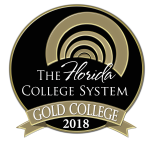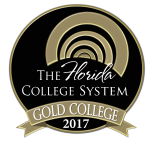MAC1140

## Precalculus Algebra

Course Code:
MAC1140

Credit Hours:
3

Effective beginning:
2023-24

Sections:
001, 002, 700

Course Description:
Topics for this course include review of algebraic techniques or operations, radicals, exponents, complex numbers, absolute value, linear and quadratic equations and inequalities, exponential and logarithmic functions, simultaneous equations and inequalities, roots of polynomials, matrices, determinants, applications, mathematical proof techniques, mathematical induction, binomial theorem, sequences and series. A “C” grade or higher must be earned to advance to a higher level mathematics course or to satisfy part of the education requirements in mathematics.

### Course Details

##### Prerequisites:

Successful completion of the equivalent of one year of high school Algebra I and one year of high school Algebra II and an acceptable score on a mathematics placement test or a “C” grade or higher in MAC 1105 or consent of the department. MAC 1114 may be taken concurrently with MAC 1140.

##### Instructors:

Stephanie Ward
wards@chipola.edu

Lisa Purvee
purveel@chipola.edu

##### Required textbooks/ course materials:

PreCalculus, Lumen Learning (OHM Generic Course Activation, ISBN-9781640871632

##### Assignment/course outline:

See first-day handout.

##### Discipline-level learning outcomes:

Demonstrate Basic Mathematical Skills and Knowledge

M-1  Solve mathematical problems by using arithmetic, algebraic, or geometric skills.

M-2  Translate basic mathematical information verbally, numerally, graphically, or symbolically.

M-3  Solve mathematical problems using appropriate technology.

M-4  Interpret mathematical models such as formulas, graphs, tables or schematics.

M-5  Solve contextual problems using mathematical processes.

##### Course-level learning outcomes:

Course-level student learning outcomesDiscipline-level learning outcomesAssessment methods

Recognize major conic sections (parabolas, hyperbolas, and ellipses) graphically and algebraically.

Solve systems of linear and nonlinear equations or inequalities in two or three variables algebraically and by using matrices.

Solve linear, quadratic, higher order polynomial, exponential, and logarithmic equations.

Perform operations with complex numbers.

Examine arithmetic and geometric series and sequences using appropriate formulas and notation.

Compute coefficients of a binomial expansion using the binomial theorem.

Prove formulas using mathematical induction.

M1, M2, M4, M5

M1, M2, M3, M4, M5

M1, M2, M3, M4, M5

M1, M2

M1, M2

M1, M2, M5

M1, M2, M3, M4, M5

Homework, Unit Tests, Cumulative Final

##### Means of accomplishing learning outcomes:

Teacher Facilitated: The teacher will be leading class discussions on the material contained in the text during each class period.

Student-centered: The students will take notes and practice solving problems during each class period.

Office Hours: The instructor will be available during office hours for individual assistance. The instructor’s schedule can be found posted on their office door and/or via their individual web site.

ACE Lab Tutors: Student tutors are available in the lab to provide individualized help. Hours can be found posted each semester on the lab door and/or via the web site.

### College-wide policies and resources

For more specific information on Chipola's college-wide academic policies and resources available to students, visit the link below.Chipola College
3094 Indian Circle
Marianna, FL 32446
850.526.2761